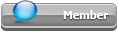## Featured Articles

Check out the latest featured articles.## File Library0# Size Of A Hydrocracker

hydrocracker sizing high-pressure 3 phases volumetric flow

3 replies to this topic
|

### #1Geetanjali

Geetanjali

Veteran Member

•• Members
•• 34 posts

Posted 27 September 2022 - 02:56 PM

Hello members,

I have a query. I need to size a hydrocracker for economic estimations.

The hydrocracker receives melted plastic stream = 10,000 kg/hr

Catalyst = 714 kg/h

Hydrogen = inlet = 1228 kg/h, outlet = 600 kg/hr, actual consumed = 628 kg/hr [ Note, I am giving a stochiometric excess of H2]

Other conditions: T = 280 C, P = 30 bar, residence time = 16 hr

My approach to size the reactor:

Vol of reactor = Volumetric flow x residence time

Volumetric flow of outlet stream = (mass of plastic/density + mass of catalyst/density + mass of hydrogen/density)   {eq.1}

At this pressure, hydrogen density is 1.5 kg/m3

Eq. 1 becomes = (10000/925 + 714/873 + 600/1.5184) = 425 m3

So, the overall volumetric flow rate = 425 m3

For 16 hr residence time = 402 x 16 = 6445 m3/hr

If I select a reactor that has a length = 9.5 m and height = 47.5 m, the volume of such a reactor would be 3365 m3

Therefore, the number of reactor I need = 6445/3365 = 2.

My question is: Is my approach correct? Should I include Hydrogen is eq. 1 or not? or should I only take liquid and solid volumes while calculating size of reactor?

I am calculating the cost from Aspen Economic analyzer.

This will be a huge help for me.

Thanks,

Geetanjalli

Edited by Geetanjali, 27 September 2022 - 04:12 PM.

### #2Bobby Strain

Bobby Strain

Gold Member

•• Members
•• 3,361 posts

Posted 27 September 2022 - 03:45 PM

None of this makes sense.

Bobby

### #3Geetanjali

Geetanjali

Veteran Member

•• Members
•• 34 posts

Posted 27 September 2022 - 04:04 PM

To size a reactor that has a flow rate of approximately 11,942 kg/hr (10,000 kg/hr liquid (highly viscous), 714 kg/hr solid, and 1228 kg/hr gas), Is my approach correct to calculate the volume of reactor?

Volumetric flow rate = (mass of liquid/density + mass of solid/density + mass of gas/density)   {eq.1}

Else, could you please suggest what type of reactor is suitable for such a process and how to size it?

Thank you,

Geetanjali

Edited by Geetanjali, 27 September 2022 - 04:10 PM.

### #4SilverShaded

Gold Member

•• Members
•• 226 posts

Posted 30 September 2022 - 10:43 AM

You need to know what space velocity you need to maintain conversion and catalyst life.

### Similar Topics